TWiki>Main Web>MatiasAlejandroBonaventura>TDAQSimulation>TDAQSimulationGroupCommunication>TDAQSimulationHybridModeling>TDAQSimulations_hybrid_continousQueueModel (2017-05-09, unknown)

# Continuous Queue model

## Fluid Equations

Almost any paper models continous queues in the same way (Towsley [ 98, 2000, 2004], Ohsaki [ 1, 2], Jason Liu [ 2006,2014], etc. This is a very trivial and intuitive way of thinking about a fluid queue: what goes in minus what goes out. The same exact model can also represent the well-known reservoir model.
Although this model is widely used it imposes the challenge of boundary detection (the queue should not go above the MAX limit, nor below the MIN limit). Depending on the integration method there are several ways to tackle boundary detection, but for method which rely on discrete time steps it usually involves backtracking or approximated boundaries detection . With QSS the boundary detection is straight forward and exact.(1)

where C is the speed at which packets are dequeued (outgoing link speed associated with the queue) a fixed constant set as parameter, and A(t) is the total arrival rate (speed at which packets are queued) which changes over time.

But this equation is still missing some important aspects:

1. The queue size shall never drop below zero
2. The queue size usually has an upper limit. The queue shall not grow above this size, and if the maximum is reach packets should be dropped
3. In some specific queues (e.g. RED queues), drops happen even before thelimit is reached so these drops should be taken into consideration in the queueSize equation.

For these considerations papers differ a little bit. Most paper don't take ALL conditions into consideration. In any case the first 2 conditions are boundary conditions must be specially handled by usual integration methods.

For the 1st condition, some papers use a conditional function in the capacity (C) term (e.g., to express that the egress of packets at speed C has to be considered only when the queue is not empty). Others just use a boundary conditions to handle 1 and 2.

For the 3rd condition, the discard probability (p(t)) has to be taken into account (which is specified by an external equation, for example for RED).

So the equation turns into:(2)

boundary condition:## Implementation in PowerDEVS

### Simple model (equation 1)

A simple implementation of equation (1) can be obtained as follows: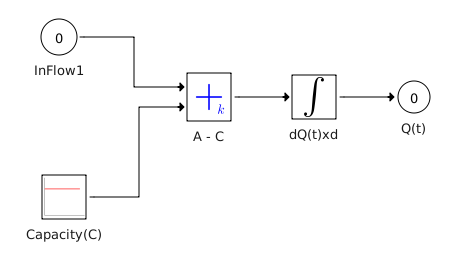#### Tests

We verify the implementation with 3 different tests:

1. A constant input rate higher than the capacity.
Expected output: queue should grow indefinetly.
2. A square input rate: with top rate higher that C, and the bottom rate=0 long enoght to allow the queue to empty.
3. A step wise input rate: start in 0, in t=5' send more than the capacity, in t=7' send less, etc

The complete top model to test the continous queue. We record input rate and queueSize (both sampled and unsampled)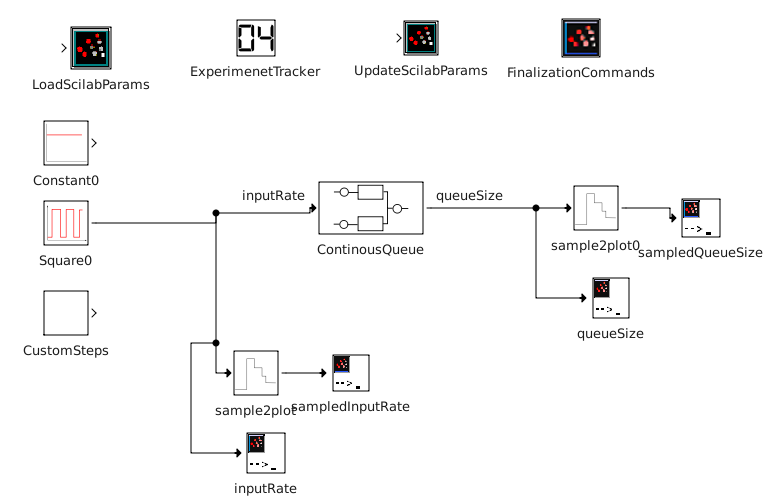Test 1: constant input rate

Parameters:

linkCapacity = 100 * M; // (bits/s)

With an inputRate=200Mb/s and a linkCapacity=100Mb/s, We expect the queue to grow at a speed of 100Mb/s.
The plot shows that at T=1s the queue is 100Mb. After 10s, the queue is 1Tb. Here we see that the queue has no boundaries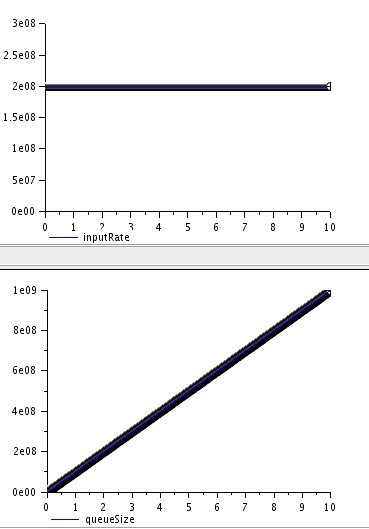Test 2: square input rate

Parameters:

linkCapacity = 100 * M; // (bits/s)
StepGenFrec = 1/2

Generating traffic at 300Mb/s the queue grows at 200Mb/s. For example from T=0 to T=1' the queue grows from 0Mb to 200Mb.
When generation stops, the queue decreases at 100Mb/s. For example from T=1' to T=2' the queue shinks from 200Mb to 100Mb.

It is interesting to see the raw ouput of the queueSize (not sampled) that generates only 10 points (when input rate changes). This is because of how QSS works.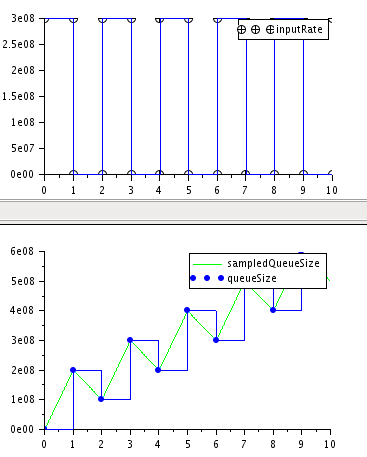step wise input rate:

Parameters:

linkCapacity = 100 * M; // (bits/s)
stepTimeChanges = [0, 1, 2, 3];

(T=0 to T=1) Here we see how the queue decreases size at 100Mb/s as there is no input. It goes below 0 Here we see that the queue has no boundaries

(T=1 to T=2) Here we see how the queue starts growing at 100Mb/s when the input rate is 200Mb/s
(T=2 to T=3) dequeue happens at linkCapacity speed (100Mb/s) as there is not more input.
(T=3 to T=4) inputRate=linkCapacity, so the queue stays constant.
(T=4 to T=5) dequeue at 100Mb/s. Here we see that the queue has no boundaries
(T=5 to T=10) queue at 100Mb/s. Here we see that the queue has no boundaries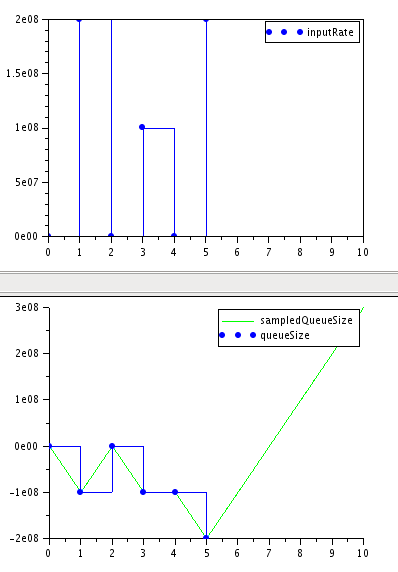### Queue model with boundary conditions

As shown in the previous tests boundaries are necesary to avoid the queueSize to go below 0 and above maxQueueSize.
Different approaches to implement boudary conditions in the integrator were tested and summaried in How to implement boundary conditions in PowerDEVS QSS.

Here we only present tests with the Final solution: Mimic logical operators (AND & OR) . It uses logical operators to set q'(t)=0 when the boudaries are reached and allow q'(t)=A-C when going out of the boundaries.

To implement boundaries eq 1 is used for q'(t) but adding q'_b(t) for the boundary conditions as follows: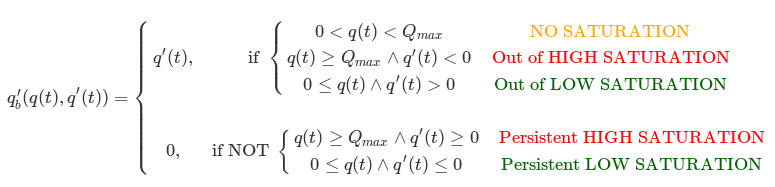Implemented in powerdevs as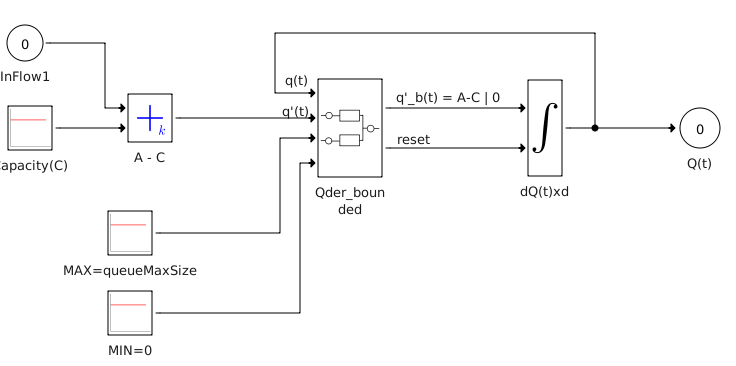#### Tests with step wise inputs

We set linkCapacity = 100 ; // (bits/s) ; queueMaxSize = 100 ; // (in bits)

We want to test several conditions:

1. Low boundary: the queue show not go below 0 when:
1. q(t) = 0 and A<C
2. q(t) > 0 and A<C
3. q(t) = 0 and A=C
2. Out of low boundary: when q(t)=0 and A>C
3. High boundary: the queue show not go above 100 when:
1. q(t) = MAX and A>C
2. q(t) < MAX and A>C
3. q(t) = MAX and A=C
4. Out of high boundary: when q(t)=MAX and A<C
5. Stability: the queue should keep its value when A=C
1. Go out of stability when A>C
2. Go out of stability when A<C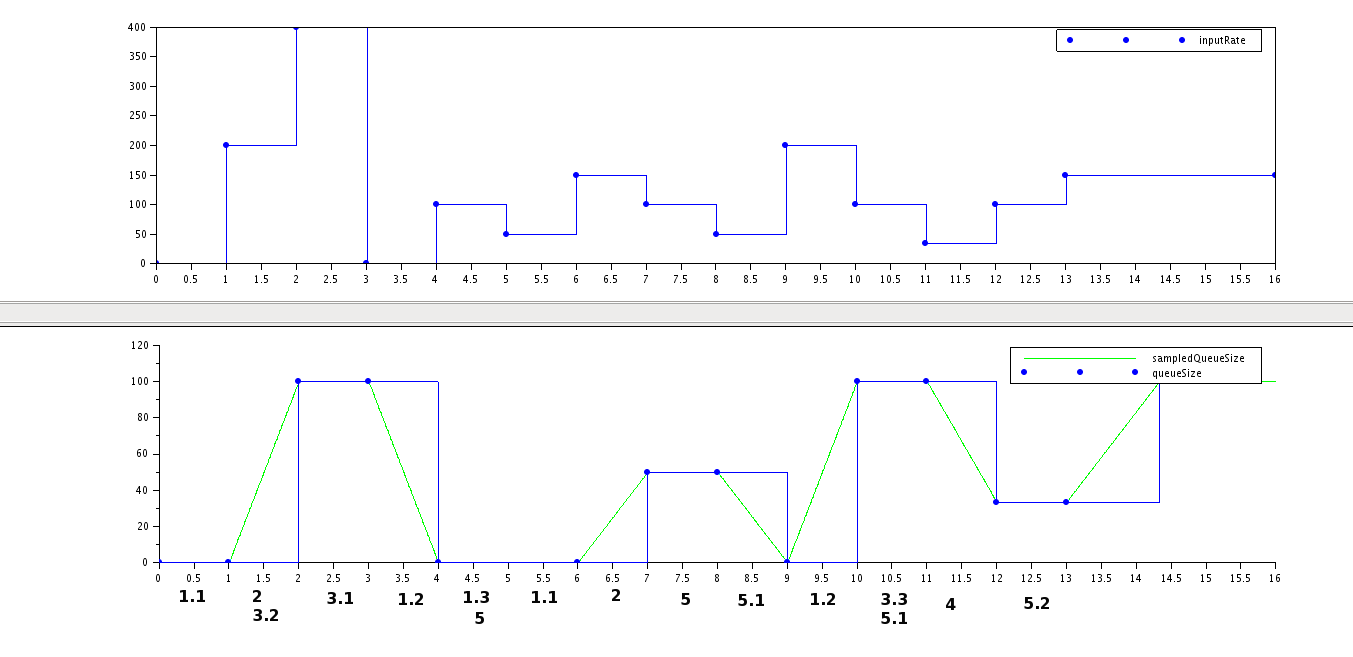We verified that 0 <= queueSize <= MAX at all moments in time.

# New Generic features added to PowerDEVS

As a result of this new generic features were added to PowerDEVS:

1. A new (coupled) model was implemented which, in conyection with the QSSIntegrator, implements a bounded integrator.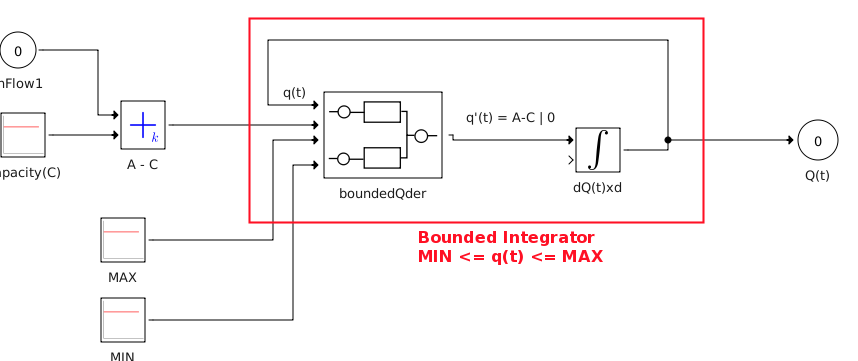2. A new library of models that implement logic and comparison operators: >, >=, <, <=, AND, OR, NOT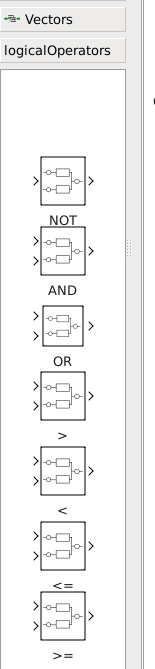3. A new atomic model that detects crossings. It mimics the cross_detect model only that the output value is not fixed, the output value is received by an input port. This model is used here to detect and set the reset port of the integrator.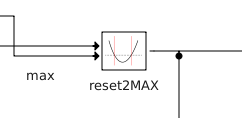-- MatiasAlejandroBonaventura - 2017-03-27

TWiki LatexModePlugin error messages:
Error! multiple equation labels 'eqn:one' defined. (Eqns. 1 and 2)
Topic revision: r6 - 2017-05-09 - unknownLog In

Webs

Welcome GuestCern SearchTWiki SearchGoogle Search Main All websCopyright &© 2008-2019 by the contributing authors. All material on this collaboration platform is the property of the contributing authors.
Ideas, requests, problems regarding TWiki? Send feedback